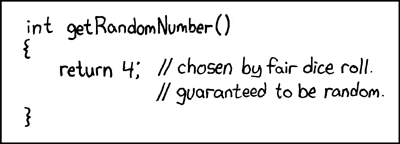## biased sample!

Posted in Statistics with tags , , , , , , , , , , , on May 21, 2019 by xi'anA chance occurrence led me to this thread on R-devel about R sample function generating a bias by taking the integer part of the continuous uniform generator… And then to the note by Kellie Ottoboni and Philip Stark analysing the reason, namely the fact that R uniform [0,1) pseudo-random generator is not perfectly continuously uniform but discrete, by the nature of numbers on a computer. Knuth (1997) showed that in this case the range of probabilities is larger than (1,1), the largest range being (1,1.03). As noted in the note, exploiting directly the pseudo-random bits of the pseudo-random generator. Shocking, isn’t it!  A fast and bias-free alternative suggested by Lemire is available as `dqsample::sample`

As an update of June 2019, sample is now fixed.

## RNG impact on MCMC [or lack thereof]

Posted in Books, R, Statistics, Travel, University life with tags , , , , , , , on July 13, 2017 by xi'anFollowing the talk at MCM 2017 about the strange impact of the random generator on the outcome of an MCMC generator, I tried in Montréal airport the following code on the banana target of Haario et al. (1999), copied from Soetaert and Laine and using the MCMC function of the FME package:

```library(FME)
Banana <- function (x1, x2) {
return(x2 - (x1^2+1)) }
pmultinorm <- function(vec, mean, Cov) {
diff <- vec - mean
ex <- -0.5*t(diff) %*% solve(Cov) %*% diff
rdet <- sqrt(det(Cov))
power <- -length(diff)*0.5
return((2.*pi)^power / rdet * exp(ex)) }
BananaSS <- function (p) {
P <- c(p, Banana(p, p))
Cov <- matrix(nr = 2, data = c(1, 0.9, 0.9, 1))
N=1e3
ejd=matrix(0,4,N)
RNGkind("Mars")
for (t in 1:N){
MCMC <- modMCMC(f = BananaSS, p = c(0, 0.7),
jump = diag(nrow = 2, x = 5), niter = 1e3)
ejd[1,t]=mean((MCMC\$pars[-1,2]-MCMC\$pars[1,2])^2)}
```

since this divergence from the initial condition seemed to reflect the experiment of the speaker at MCM 2017. Unsurprisingly, no difference came from using the different RNGs in R (which may fail to contain those incriminated by the study)…

## a grim knight [cont’d]

Posted in Books, Kids, pictures, R, Statistics with tags , , , , , , , on October 20, 2016 by xi'anAs discussed in the previous entry, there are two interpretations to this question from The Riddler:

“…how long is the longest path a knight can travel on a standard 8-by-8 board without letting the path intersect itself?”as to what constitutes a path. As a (terrible) chess player, I would opt for the version on the previous post, the knight moving two steps in one direction and one in the other (or vice-versa), thus occupying three squares on the board. But one can consider instead the graph of the moves of that knight, as in the above picture and as in The Riddler. And since I could not let the problem go I also wrote an R code (as clumsy as the previous one!) to explore at random (with some minimal degree of annealing) the maximum length of a self-avoiding knight canter.The first maximal length I found this way is 32, although I also came by hand to a spiralling solution with a length of 33.Running the R code longer over the weekend however led to a path of length 34, while the exact solution to the riddle is 35, as provided by the Riddler (and earlier in many forms, including Martin Gardner’s and Donald Knuth’s).[An unexpected side-effect of this riddle was ending up watching half of Bergman’s Seventh Seal in Swedish…]